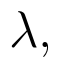Select Page

# MCQ in English Answers for CBSE Maths 12 Science Vector Algebra

MCQ in English Answers for CBSE Maths 12 Science Vector Algebra to enable students to get Answers in a narrative video format for the specific question.

Expert Teacher provides MCQ Answers for CBSE Maths 12 Science Vector Algebra through Video Answers in English language. This video solution will be useful for students to understand how to write an answer in exam in order to score more marks. This teacher uses a narrative style for a question from Vector Algebra not only to explain the proper method of answering question, but deriving right answer too.

Please find the question below and view the Answer in a narrative video format.

Question:

## Similar Questions from CBSE, 12th Science, Maths, Vector Algebra

Question 1 : Find the unit vector in the direction ofifand(View Answer Video)

Question 2 : Find the value ofif the points with position vectorsandare coplanar.  (View Answer Video)

Question 3 :  Write a unit vector in the direction of the sum of vectorsand(View Answer Video)

Question 4 : Find the angle between the vectorsand(View Answer Video)

Question 5 : Find a unit vector parallel to the sum of vectorsand(View Answer Video)

### Application of Integrals

Question 1 : Find the area of the region. (View Answer Video)

Question 2 : Find the area of the region bounded by the curvesand x = 3. (View Answer Video)

Question 3 : A farmer has a field of shape bounded byand x = 3, he wants to divide this into his two sons equally by a straight line x = c. Can you find c? What value, you find in the person ? (View Answer Video)

Question 4 : Using integration, find the area of the triangle formed by a positive x-axis and tangent and normal to the circleat. (View Answer Video)

Question 5 : Find the area of the smaller region bounded by the ellipseand the straight line 8x + 3y = 12. (View Answer Video)

### Inverse Trigonometric Functions

Question 1 : Write the principal value of(View Answer Video)

Question 2 :

Write the value of the following:Question 3 :then find the value of x. (View Answer Video)

Question 4 :is equal to :

Question 5 : Using principal values, write the value of(View Answer Video)

### Determinant

Question 1 : Evaluate. (View Answer Video)

Question 2 : If A is a symmetric matrix, then? (View Answer Video)

Question 3 : The solution of the following system of equation is 2x + 3y = 5, 5x – 2y = 3. (View Answer Video)

Question 4 : If a, b, c are in AP, then determinant. (View Answer Video)

Question 5 : Let, where. THhen (View Answer Video)